### Python SciPy Interpolation

Python Scipy Interpolation

What is Interpolation?
Interpolation is a useful mathematical and statistical tool used to estimate values between two points.It is the process of finding a value between two points on a line or a curve.

scipy.interpolate in python:

Let us create some data and see how this interpolation can be done using the scipy.interpolate package.

```
import numpy as np
from scipy import interpolate
import matplotlib.pyplot as plt
x = np.linspace(0, 4, 12)
y = np.cos(x**2/3+4)
print x,y

```

The above program will generate the following output.

```(
array([0.,  0.36363636,  0.72727273,  1.09090909,  1.45454545, 1.81818182,
2.18181818,  2.54545455,  2.90909091,  3.27272727,  3.63636364,  4.]),

array([-0.65364362,  -0.61966189,  -0.51077021,  -0.31047698,  -0.00715476,
0.37976236,   0.76715099,   0.99239518,   0.85886263,   0.27994201,
-0.52586509,  -0.99582185])
)
```

Now, we have two arrays. Assuming those two arrays as the two dimensions of the points in space, let us plot using the following program and see how they look like.

```plt.plot(x, y,%u2019o%u2019)
plt.show()

```

The above program will generate the following output.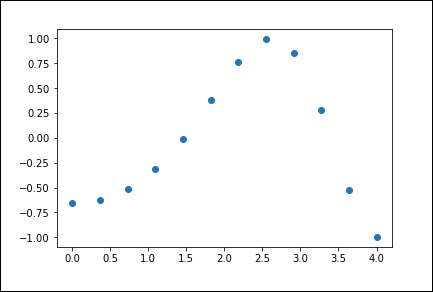# scipy.interpolate.interp1d

The interp1d class in the scipy.interpolate is a convenient method to create a function based on fixed data points, which can be evaluated anywhere within the domain defined by the given data using linear interpolation.

By using the above data, let us create a interpolate function and draw a new interpolated graph.

```f1 = interp1d(x, y,kind = 'linear')

f2 = interp1d(x, y, kind = 'cubic')```

Using the interp1d function, we created two functions f1 and f2. These functions, for a given input x returns y. The third variable kind represents the type of the interpolation technique. 'Linear', 'Nearest', 'Zero', 'Slinear', 'Quadratic', 'Cubic' are a few techniques of interpolation.

Now, let us create a new input of more length to see the clear difference of interpolation. We will use the same function of the old data on the new data.

```

xnew = np.linspace(0, 4,30)
plt.plot(x, y, 'o', xnew, f(xnew), '-', xnew, f2(xnew), '--')
plt.legend(['data', 'linear', 'cubic','nearest'], loc = 'best')
plt.show()

```

`The above program will generate the following output.`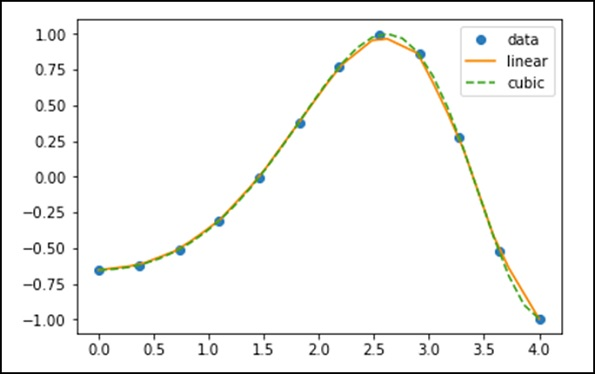## Splines

To draw smooth curves through data points, drafters once used thin flexible strips of wood, hard rubber, metal or plastic called mechanical splines. To use a mechanical spline, pins were placed at a judicious selection of points along a curve in a design, and then the spline was bent, so that it touched each of these pins.

Clearly, with this construction, the spline interpolates the curve at these pins. It can be used to reproduce the curve in other drawings. The points where the pins are located is called knots. We can change the shape of the curve defined by the spline by adjusting the location of the knots.

### Univariate Spline

One-dimensional smoothing spline fits a given set of data points. The UnivariateSpline class in scipy.interpolate is a convenient method to create a function, based on fixed data points class %u2013 scipy.interpolate.UnivariateSpline(x, y, w = None, bbox = [None, None], k = 3, s = None, ext = 0, check_finite = False).

Parameters %u2212 Following are the parameters of a Univariate Spline.

• This fits a spline y = spl(x) of degree k to the provided x, y data.

• %u2018w%u2019 %u2212 Specifies the weights for spline fitting. Must be positive. If none (default), weights are all equal.

• %u2018s%u2019 %u2212 Specifies the number of knots by specifying a smoothing condition.

• %u2018k%u2019 %u2212 Degree of the smoothing spline. Must be <= 5. Default is k = 3, a cubic spline.

• Ext %u2212 Controls the extrapolation mode for elements not in the interval defined by the knot sequence.

• if ext = 0 or %u2018extrapolate%u2019, returns the extrapolated value.

• if ext = 1 or %u2018zero%u2019, returns 0

• if ext = 2 or %u2018raise%u2019, raises a ValueError

• if ext = 3 of %u2018const%u2019, returns the boundary value.

• check_finite %u2013 Whether to check that the input arrays contain only finite numbers.

Let us consider the following example.

```import matplotlib.pyplot as plt
from scipy.interpolate import UnivariateSpline
x = np.linspace(-3, 3, 50)
y = np.exp(-x**2) + 0.1 * np.random.randn(50)
plt.plot(x, y, 'ro', ms = 5)
plt.show()```

Use the default value for the smoothing parameter.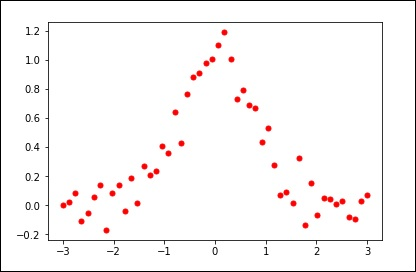```spl = UnivariateSpline(x, y)
xs = np.linspace(-3, 3, 1000)
plt.plot(xs, spl(xs), 'g', lw = 3)
plt.show()

```

Manually change the amount of smoothing.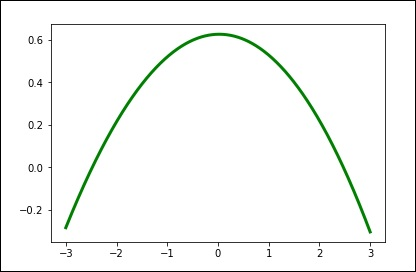```
spl.set_smoothing_factor(0.5)
plt.plot(xs, spl(xs), 'b', lw = 3)
plt.show()

```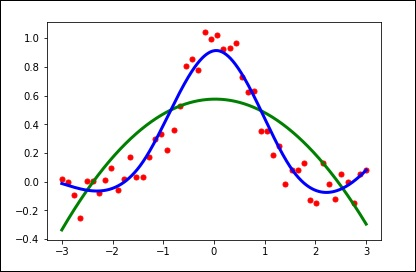As listed below, this sub-package contains spline functions and classes, one-dimensional and multi-dimensional (univariate and multivariate) interpolation classes, Lagrange and Taylor polynomial interpolators, and wrappers for FITPACK and DFITPACK functions.

## Univariate interpolation

 `interp1d`(x, y[, kind, axis, copy, %u2026]) Interpolate a 1-D function. `BarycentricInterpolator`(xi[, yi, axis]) The interpolating polynomial for a set of points `KroghInterpolator`(xi, yi[, axis]) Interpolating polynomial for a set of points. `PchipInterpolator`(x, y[, axis, extrapolate]) PCHIP 1-d monotonic cubic interpolation. `barycentric_interpolate`(xi, yi, x[, axis]) Convenience function for polynomial interpolation. `krogh_interpolate`(xi, yi, x[, der, axis]) Convenience function for polynomial interpolation. `pchip_interpolate`(xi, yi, x[, der, axis]) Convenience function for pchip interpolation. `Akima1DInterpolator`(x, y[, axis]) Akima interpolator `CubicSpline`(x, y[, axis, bc_type, extrapolate]) Cubic spline data interpolator. `PPoly`(c, x[, extrapolate, axis]) Piecewise polynomial in terms of coefficients and breakpoints `BPoly`(c, x[, extrapolate, axis]) Piecewise polynomial in terms of coefficients and breakpoints.

## Multivariate interpolation

Unstructured data:

 `griddata`(points, values, xi[, method, %u2026]) Interpolate unstructured D-dimensional data. `LinearNDInterpolator`(points, values[, %u2026]) Piecewise linear interpolant in N dimensions. `NearestNDInterpolator`(x, y) Nearest-neighbour interpolation in N dimensions. `CloughTocher2DInterpolator`(points, values[, tol]) Piecewise cubic, C1 smooth, curvature-minimizing interpolant in 2D. `Rbf`(*args) A class for radial basis function approximation/interpolation of n-dimensional scattered data. `interp2d`(x, y, z[, kind, copy, %u2026]) Interpolate over a 2-D grid.

For data on a grid:

 `interpn`(points, values, xi[, method, %u2026]) Multidimensional interpolation on regular grids. `RegularGridInterpolator`(points, values[, %u2026]) Interpolation on a regular grid in arbitrary dimensions `RectBivariateSpline`(x, y, z[, bbox, kx, ky, s]) Bivariate spline approximation over a rectangular mesh.

Tensor product polynomials:

 `NdPPoly`(c, x[, extrapolate]) Piecewise tensor product polynomial

## 1-D Splines

 `BSpline`(t, c, k[, extrapolate, axis]) Univariate spline in the B-spline basis. `make_interp_spline`(x, y[, k, t, bc_type, %u2026]) Compute the (coefficients of) interpolating B-spline. `make_lsq_spline`(x, y, t[, k, w, axis, %u2026]) Compute the (coefficients of) an LSQ B-spline.

Functional interface to FITPACK routines:

 `splrep`(x, y[, w, xb, xe, k, task, s, t, %u2026]) Find the B-spline representation of 1-D curve. `splprep`(x[, w, u, ub, ue, k, task, s, t, %u2026]) Find the B-spline representation of an N-dimensional curve. `splev`(x, tck[, der, ext]) Evaluate a B-spline or its derivatives. `splint`(a, b, tck[, full_output]) Evaluate the definite integral of a B-spline between two given points. `sproot`(tck[, mest]) Find the roots of a cubic B-spline. `spalde`(x, tck) Evaluate all derivatives of a B-spline. `splder`(tck[, n]) Compute the spline representation of the derivative of a given spline `splantider`(tck[, n]) Compute the spline for the antiderivative (integral) of a given spline. `insert`(x, tck[, m, per]) Insert knots into a B-spline.

Object-oriented FITPACK interface:

 `UnivariateSpline`(x, y[, w, bbox, k, s, ext, %u2026]) One-dimensional smoothing spline fit to a given set of data points. `InterpolatedUnivariateSpline`(x, y[, w, %u2026]) One-dimensional interpolating spline for a given set of data points. `LSQUnivariateSpline`(x, y, t[, w, bbox, k, %u2026]) One-dimensional spline with explicit internal knots.

## 2-D Splines

For data on a grid:

 `RectBivariateSpline`(x, y, z[, bbox, kx, ky, s]) Bivariate spline approximation over a rectangular mesh. `RectSphereBivariateSpline`(u, v, r[, s, %u2026]) Bivariate spline approximation over a rectangular mesh on a sphere.

For unstructured data:

 `BivariateSpline` Base class for bivariate splines. `SmoothBivariateSpline`(x, y, z[, w, bbox, %u2026]) Smooth bivariate spline approximation. `SmoothSphereBivariateSpline`(theta, phi, r[, %u2026]) Smooth bivariate spline approximation in spherical coordinates. `LSQBivariateSpline`(x, y, z, tx, ty[, w, %u2026]) Weighted least-squares bivariate spline approximation. `LSQSphereBivariateSpline`(theta, phi, r, tt, tp) Weighted least-squares bivariate spline approximation in spherical coordinates.

##Article contributed by Yashwanth Gudigamolla#### More Articles of yashwanth gudigamolla:

Name Views Likes
Python SciPy stats.skew() function 1588 17
Python Scipy Stats 1167 14
Python SciPy stats beta() function 2029 14
Python Scipy Special (List of Different Functions) 1548 15
Python SciPy Integration 1409 12
Python Scipy Fourier Transforms(scipy.fftpack) 1462 17
Python Scipy linalg 1316 13
Python SciPy stats.expon() function 2287 17
Python SciPy stats.chi() function 993 24
Python Scipy Multidimentional image processing (scipy.ndimage) 1618 26
SciPy - ODR 1485 23
Python SciPy stats.cosine() function 958 14
Python SciPy stats.trim1() function 1051 11
Python SciPy stats.tvar() function 1107 16
Python SciPy stats.tvar() function 1046 23
SciPy - Basic Functionality 1152 27
Python SciPy stats.betaprime() function 1006 13
Python Scipy CSGraph 1204 19
Python Scipy File Input and Output (IO) 1616 20
Python Scipy Optimize 1444 23
Python Scipy Special package (scipy.special) 1107 25
Python SciPy stats.tsem() function 949 12
Python SciPy stats.variation() function 1386 16
Python SciPy stats.tsem() function 998 14
SciPy - Spatial 1179 25
Python program to insert an element into binary search tree 9696 29
Python SciPy stats.chi2() function 1905 18
Python SciPy Interpolation 6650 21
C++ program to find inorder predecessor and successor for a given key in binary search tree with recursion 2478 15
python program to check if two trees are identical using recursion 1192 12
Python SciPy constants 2014 18
Python SciPy stats.sem() function 1338 22
Python Scipy Introduction 1684 26
Python SciPy stats alpha() function 1170 23
SciPy - Cluster 1285 20